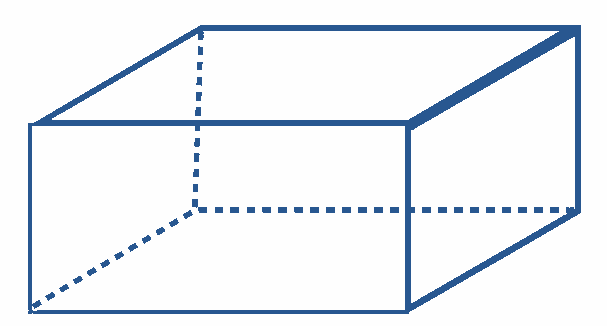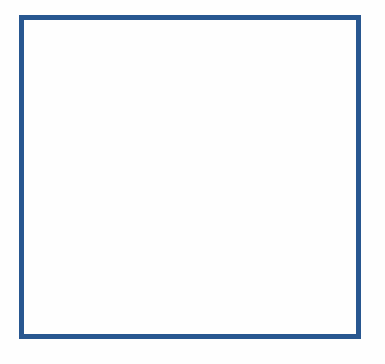The top view of the below shape is

# The top view of the below shape is1. A
Rectangle
2. B
Square
3. C
Triangle
4. D
Circle

Fill Out the Form for Expert Academic Guidance!l

+91

Live ClassesBooksTest SeriesSelf Learning

Verify OTP Code (required)

### Solution:

The given figure isThe top view of the given shape isWe can observe that the top view is a rectangle.
The correct option is (1).

## Related content

 Important Topic of Physics: Reynolds Number Distance Speed Time Formula Refractive Index Formula Mass Formula Electric Current Formula Electric Power Formula Resistivity Formula Weight Formula Linear Momentum Formula Non Contact Force+91

Live ClassesBooksTest SeriesSelf Learning

Verify OTP Code (required)